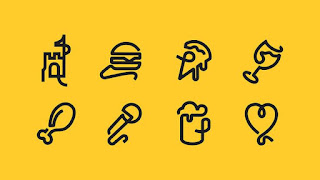#INDEX
• Introduction to problem
• Algorithm
• Flowchart
• Program in C language
• Output

## Introduction to Program

In this problem statement, we have three number and out of these three number we have to find, which is bigger or largest. this can be achieve by comparing these three number with each other.

## Step1: declare three variable named A, B and C

Step2: Read the value of A, B and C from user

Step3: if A>B then
if A > C then
print A is greater
otherwise
print C is greater
otherwise
if B > C then
print B is greater
otherwise
print C is greater
Step4: Exit

## Program in C language

#include<stdio.h>
#include<conio.h>
void main()
{
int num1,num2,num3;
printf("enter any three number\n");
scanf("%d %d %d",&num1,&num2,&num3);
if(num1>num2)
{
if(num1>num3)
{
printf("%d is greater than %d ,%d",num1,num2,num3);
}
else
{
printf("%d is greater than %d ,%d",num3,num1,num2);
}
}
else
{
if(num2>num3)
{
printf("%d is greater than %d ,%d",num2,num1,num3);
}
else
{
printf("%d is greater than %d ,%d",num3,num1,num2);
}
}
}

## OutPut

enter any three number
23
3
24

24 is greater than 23, 3